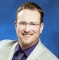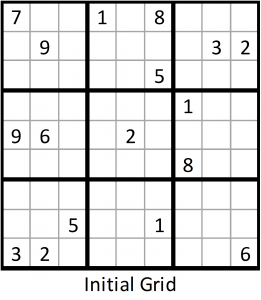Part 1 of this topic presented a simple Sudoku solver. By treating Sudoku as an exact cover problem, the algorithm efficiently found solutions to simple Sudoku problems using basic logic. Unfortunately, the simple solver fails when presented with more difficult Sudoku problems. The puzzle on the right was obtained from Sudoku Garden (http://sudokugarden.de/) and is reproduced here in its original form under the creative commons attributions license. The basic solver manages to solve the majority of the puzzle before giving up. This post demonstrates how the entire puzzle can be solved through a combination of the simple solver from Part 1 and an adaptation of a backtracking algorithm called Algorithm X. The solver can also solve the world’s hardest Sudoku puzzles, but those puzzles are not reproduced here due to copyright.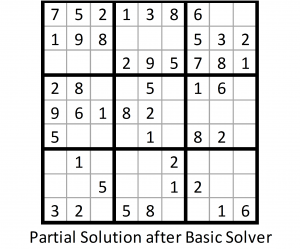Algorithm X is an efficient backtracking algorithm for solving exact cover problems (Knuth, 2009). The algorithm forms a search tree with columns of the exact cover matrix forming the nodes of the tree and rows of the exact cover matrix forming the branches of the tree. The algorithm navigates the search tree until a solution is found, and then terminates. The adaptation of Algorithm X used in this post is a bit convoluted, so is only described in the context of the example puzzle above.

Running the basic solver on the puzzle at the beginning of this post yields the grid on the right. Having failed to obtain a solution using the basic solver, the IML program then calls my modified version of Algorithm X. The search tree formed by the algorithm is shown below. Note that this tree is a graphical representation of the process taken by the algorithm, and is not generated by the IML code.

The algorithm begins by picking a column in the exact cover matrix with a sum greater than one but as small as possible (preferably a sum of two). That is, the algorithm chooses a constraint that is not satisfied, but which has the smallest number of candidate rows. In the case of the current puzzle, column 122 is chosen. Column 122 corresponds to the constraint that row 5 must contain the number 5. Two rows are candidates to satisfy the condition: row 392 (which corresponds to a 5 in row 5 column 8) and row 401 (which corresponds to a 5 in row 5 column 9). Selecting the first candidate row, 392, and then running the basic solver yields an impossible solution. Selecting the second candidate row, 401, and running the basic solver fails to fully solve the puzzle, but moves us closer to the solution.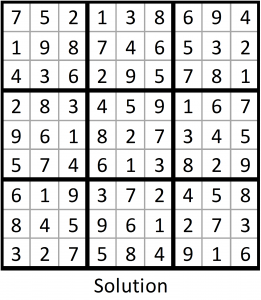Column 323 (which corresponds to the constraint that box 9 must contain the number 8) is chosen as the next node, as it can only be satisfied by two rows. Two rows are candidates to satisfy the condition: row 566 (which corresponds to number 8 in row 7 column 9) and row 647 (which corresponds to number 8 in row 8 column 9). Selecting the first candidate row, 566, and then running the basic solver fails to fully solve the puzzle, but moves us closer to the solution. Column 309 (which corresponds to the constraint that box 8 must contain the number 3) is chosen as the next node, as it can only be satisfied by two rows. Two rows are candidates to satisfy the condition: row 516 (which corresponds to number 3 in row 7 column 4) and row 597 (which corresponds to number 3 in row 8 column 4). Selecting the first candidate row, 516, and then running the basic solver yields the solution on the right.

Rejected Nodes

The search tree for this example was relatively simple. The algorithm tested only three nodes and four branches to find a solution. Though some rows were pruned from the tree (e.g., row 392), none of the nodes were rejected. It is possible that none of the rows will yield a solution for a particular column. For example, if selecting rows 516 and 597 both yielded impossible solutions for column 309, the node corresponding to column 309 would be pruned from the search tree.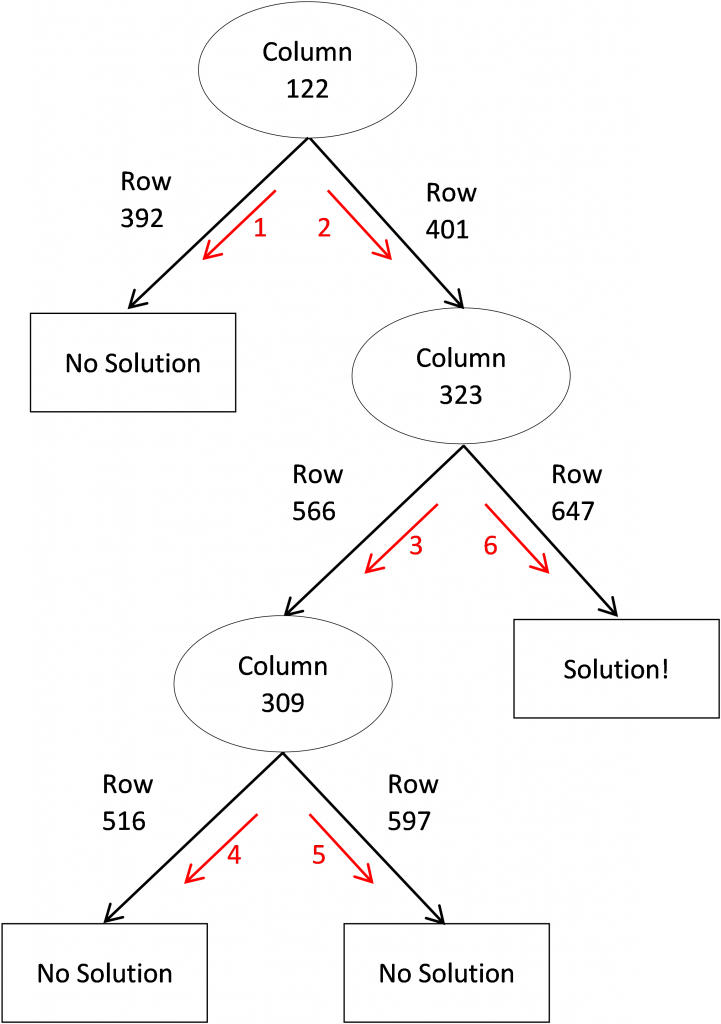After rejecting the node corresponding to column 309, the algorithm would test the next candidate row for column 323, row 647. In this hypothetical example, selecting row 647 for column 323 yields a solution.

Differences from Algorithm X

My adaptation of Algorithm X differs from the original in four important ways. First, IML does not include functionality for recursive algorithms. As a workaround, my version of Algorithm X uses a DO loop to generate the search tree. Second, Algorithm X updates the exact cover matrix by deleting rows and columns. In contrast, my version of Algorithm X updates the exact cover matrix by zeroing out cells. This is less efficient, but was done to keep the code relatively simple. Third, Algorithm X is nondeterministic, meaning that it can exhibit different behavior across different runs. In contrast, my adaptation is deterministic, allowing readers to obtain the same results that are presented in this document. Finally, Algorithm X finds all possible solutions to an exact cover problem whereas my adaptation of Algorithm X stops upon finding any solution.

References

Ercsey-Ravasz, M., & Toroczkai, Z. (2012). The chaos within Sudoku. Scientific reports, 2.

Knuth, D. E. (2009). Dancing links. Millenial Perspectives in Computer Science, 18(4).

Syntax

```proc iml; /***********************************************************************************************/ /* Module UPDATECONSTRAINTMATRIX uses basic logic to update/solve the constraint matrix */ /***********************************************************************************************/ start updateConstraintMatrix(constraintMatrix,result); change = 1; do until (change = 0); tempSum = constraintMatrix[+];   *Rows; rowSums = constraintMatrix[,+]; if (rowSums < 4)[+] > 0 then do; rowIndex = loc(rowSums < 4); constraintMatrix[rowIndex,] = 0; end;   *Columns; columnSums = constraintMatrix[+,]; if (columnSums = 1)[+] > 0 then do; columnList = loc(columnSums = 1); do i = 1 to ncol(columnList); column = columnList[i]; row = loc(constraintMatrix[,column] = 1); columnIndex = loc(constraintMatrix[row,]=1); rowindex = setdif(1:729,row); constraintMatrix[rowindex,columnIndex] = 0; end; end;   tempSumNew = constraintMatrix[+]; change = tempSumNew - tempSum; end;   *Check to see if solved; columnSums = constraintMatrix[+,]; nConstraintsSolved = (columnSums = 1)[+]; if nConstraintsSolved = 324 then result = 1;   *Check to see if impossible or just unsolved; else do; nErrors = (columnSums = 0)[+]; if nErrors > 0 then result = -1; *If impossible; else result = 0; *Otherwise mark as unsolved; end; finish;   /***********************************************************************************************/ /* Module PRINTSOLUTION prints the solution grid from the current constraint matrix */ /***********************************************************************************************/ start printSolution(constraintMatrix); solution = j(9,9,0); do i = 1 to 81; rows = (((i-1)*9 + 1):((i-1)*9 + 9)); temp = constraintMatrix[rows,i]; if (temp = 1)[+] = 1 then value = loc(temp = 1); else value = .; solution[i] = value; end; print solution; finish;           /***********************************************************************************************/ /* Module SUDOKUSOLVER creates the constraint matrix and tries to solve the puzzle first with */ /* a basic solver and then with an adaptation of Algorithm X by Donald Knuth */ /***********************************************************************************************/ start sudokuSolver(initialGrid); print "Welcome to Steve's Sudoku Solver!"; reset noname; print "Input grid:"; print initialGrid; /***********************************************************************************************/ /* Start timer and create constraint matrix */ /***********************************************************************************************/ time_initial = time();   *Create cell constraints; cellConstraints = i(81) @ j(9,1,1);   *Create row constraints; rowConstraints = i(9) @ j(9,1,1) @ i(9);   *Create column constraints; columnConstraints = j(9,1,1) @ i(81);   *Create block constraints; blockConstraints = i(3) @ j(3,1,1) @ i(3) @ j(3,1,1) @ i(9);   *Combine all constraints into single matrix; constraintMatrix = cellConstraints || rowConstraints || columnConstraints || blockConstraints;   *Add labels to constraint matrix; do row = 1 to 9; do column = 1 to 9; do candidate = 1 to 9; name = concat("r",trim(char(row,1)),"c",char(column,1),"_",char(candidate,1)); rowLabels = rowLabels // name; end; end; end;   mattrib constraintMatrix rowName = rowLabels; /***********************************************************************************************/ /* Modify constraint matrix using clues from initial grid */ /***********************************************************************************************/ do gridRow = 1 to 9; do gridColumn = 1 to 9; value = initialGrid[gridRow,gridColumn]; if value ^= . then do; *Find the row in the constraint matrix that corresponds to the specified row and column in the grid; row = (gridRow-1) * 81 + (gridColumn - 1) * 9 + value; *Find all columns where the specified row contains a value of one; columnIndex = loc(constraintMatrix[row,]=1); *In columns where specified row equals one, change all other values to zero; rowindex = setdif(1:729,row); constraintMatrix[rowindex,columnIndex] = 0; end; end; end; /***********************************************************************************************/ /* Attempt to solve Sudoku using basic logic */ /***********************************************************************************************/ result = 0; run updateConstraintMatrix(constraintMatrix,result); /***********************************************************************************************/ /* If a solution has been found, print the solution */ /***********************************************************************************************/ if result = 1 then do; print "Sudoku puzzle successfully solved."; run printSolution(constraintMatrix); end; /***********************************************************************************************/ /* If basic logic indicates that no solution is possible, print message and exit */ /***********************************************************************************************/ else if result = -1 then do; print "No possible solution."; end; /***********************************************************************************************/ /* If simple solver did not reach a complete solution but a solution still */ /* appears to be plausible, print the partial solution and begin the search algorithm. */ /***********************************************************************************************/ else do; print "Puzzle cannot be solved with basic logic."; print "The program will now begin a search algorithm to find the solution."; print "Partial solution (prior to beginning search algorithm):"; run printSolution(constraintMatrix); iteration = 0; algorithmComplete = 0; tempConstraintMatrix = ConstraintMatrix;   do until (algorithmComplete = 1); iteration = iteration + 1; rowSelected = 0; /***********************************************************************************************/ /* Begin Algorithm X */ /***********************************************************************************************/ *Determine whether any steps have been completed; *If no steps have been completed, then pick a column and row; if isempty(steps) then do; *Determine number of candidates for each constraint/column; columnSums = tempConstraintMatrix[+,];   *Sort columns from lowest to highest, except values columns containing 1 (which are last); columnSums2 = columnSums; columnSums2[loc(columnSums = 1)] = 10; call sortndx(tempOrder,columnSums2`);   *Select column with smallest number of candidates; tempColumn = tempOrder;   *Pick first row for selected column; rowCandidates = loc(tempConstraintMatrix[,tempColumn]=1); tempRow = rowCandidates;   *Create steps matrix; steps = tempColumn || tempRow || .; mattrib steps colname={column row result}; end;   *If last row was neither successful or a failure, pick a column and a row; else if steps[nrow(steps),3] = 0 then do; *Determine number of candidates for each constraint/column; columnSums = tempConstraintMatrix[+,];   *Sort columns from lowest to highest, except values columns containing 1 (which are last); columnSums2 = columnSums; columnSums2[loc(columnSums = 1)] = 10; call sortndx(tempOrder,columnSums2`);   *Select column with smallest number of candidates; tempColumn = tempOrder;   *Pick first row for selected column; rowCandidates = loc(tempConstraintMatrix[,tempColumn]=1); tempRow = rowCandidates;   *Update steps matrix; steps = steps // (tempColumn || tempRow || .); end;   *If last step was a failure try following two options; *1) Retry step with different row; *2) Go back to previous step; else if steps[nrow(steps),3] = -1 then do until (rowselected = 1); failedColumn = steps[nrow(steps),1]; failedRow = steps[nrow(steps),2];   *Update constraint matrix with row selection; tempConstraintMatrix = ConstraintMatrix; do i = 1 to nrow(steps) - 1; temprow = steps[i,2]; tempColumnIndex = loc(tempConstraintMatrix[tempRow,] = 1); tempIndex = setdif(1:729,tempRow); tempConstraintMatrix[tempIndex,tempColumnIndex] = 0; end;   tempColumn = failedColumn;   *If failed row is not the last row, move to next row; rowCandidates = loc(tempConstraintMatrix[,tempColumn]=1);   *If failed row is the last row, move back to previous step; if loc(rowCandidates = failedRow) < ncol(rowCandidates) then do; candidateNumber = loc(rowCandidates = failedRow) + 1; tempRow = rowCandidates[candidateNumber];   *Update steps matrix; steps[nrow(steps),2] = temprow; steps[nrow(steps),3] = .;   rowSelected = 1; end;   else do; steps = steps[1:nrow(steps) - 1,]; steps[nrow(steps),3] = -1; end; end;   *Update constraint matrix with column and row selection; tempConstraintMatrix = ConstraintMatrix; do i = 1 to nrow(steps); temprow = steps[i,2]; tempColumnIndex = loc(tempConstraintMatrix[tempRow,] = 1); tempIndex = setdif(1:729,tempRow); tempConstraintMatrix[tempIndex,tempColumnIndex] = 0; end;   *Iterative update of constraint matrix; run updateConstraintMatrix(tempConstraintMatrix,result); steps[nrow(steps),3] = result;   if iteration = 5000 then do; print "Maximum number of iterations reached"; run printSolution(tempConstraintMatrix); algorithmComplete = 1; end;   else if result = 1 then do; print "Success! Final solution:"; run printSolution(tempConstraintMatrix); algorithmComplete = 1; end; end;   print "Number of search iterations required:" iteration; end; jump: /***********************************************************************************************/ /* Print time elapsed and number of search iterations required */ /***********************************************************************************************/ time_elapsed = time() - time_initial; print "Elapsed time (in seconds):" time_elapsed; reset name; finish;       *Input starting grid; initialGrid = {7 . . 1 . 8 . . ., . 9 . . . . . 3 2, . . . . . 5 . . ., . . . . . . 1 . ., 9 6 . . 2 . . . ., . . . . . . 8 . ., . . . . . . . . ., . . 5 . . 1 . . ., 3 2 . . . . . . 6};     *Run solver; run sudokuSolver(initialGrid); quit;```
ShareAnalytical Training Consultant

Stephen Mistler is an analytical training consultant at SAS. He teaches multiple SAS courses, including: Determining Power and Sample Size using SAS/STAT Software, Introduction to Programming with SAS/IML Software, Multilevel Modeling of Hierarchical and Longitudinal Data, and Structural Equation Modeling using SAS. His educational background is in quantitative psychology and he is currently working on his dissertation, which focuses on the use of multiple imputation for multilevel data. Stephen was named a SAS Student Ambassador Honorable Mention for papers that he presented at SAS Global Forum. He also received a SAS Summer Fellowship in Statistical Software Development.

### Related Posts

1.•